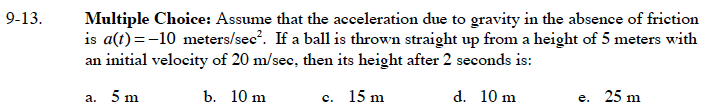### Home > CALC > Chapter 9 > Lesson 9.1.1 > Problem9-13

9-13.
1. Multiple Choice: Assume that the acceleration due to gravity in the absence of friction is a(t) = −10 meters/sec2. If a ball is thrown straight up from a height of 5 meters with an initial velocity of 20 m/sec, then its height after 2 seconds is: Homework Help ✎

1. 5 m

2. 10 m

3. 15 m

4. 10 m

5. 25 m$v(t)=\int{a(t)}dt=-10t+C$

$v(0)=20=-10(0)+C\text{ }C=20$

Evaluate h(2).